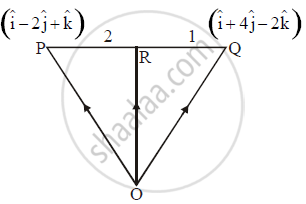# If p=i−2j+k and q=i+4j+2k are position vector (P.V.) of points P and Q, find the position vector of the point R which divides segment PQ internally in the ratio 2:1 - Mathematics and Statistics

Sum

If  bar p = hat i - 2 hat j + hat k and bar q = hat i + 4 hat j - 2 hat k are position vector (P.V.) of points P and Q, find the position vector of the point R which divides segment PQ internally in the ratio 2:1

#### Solution 1

R is the point which divides the line segment joining the points PQ internally in the ratio 2:1.

bar r=(2(bar q)+1(bar p))/(2+1)

=(2(hati+4hatj-2hatk)+1(hati-2hatj+hatk))/(3)

=(3hati+6hatj-3hatk)/3

barr=hati+2hatj-hatk

The position vector of point R is  hati+2hatj-hatk

#### Solution 2Position vector of point R in

vec(OR)=(vec(OQ)xx2+1xxvec(OP))/(2+1)

vec(OR)=(2(hati+4hatj-2hatk)+1(hati-2hatj+hatk))/3

vec(OR)=(2hati+8hatj-4hatk+hati-2hatj+hatk)/3

vec(OR)=(3hati+6hatj-3hatk)/3

vec(OR)=hati+2hatj-hatk

Concept: Section Formula
Is there an error in this question or solution?
2015-2016 (March)

Share